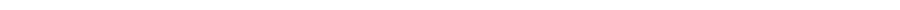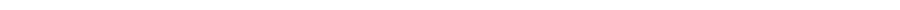Mathcounts Prep
 The goal of the camp is to encourage students' interest in math, to develop their problem solving skills, and to inspire them to excel in math competitions like Mathcounts, AMC, MOEMS, Minnesota Junior High Math League. The topics covered in this Camp are: Operations with Fractions/ Decimals/ Percents  Ratios and Proportions                        Counting Principles                        Exponents and Radicals                            Absolute Values  Geometry Similar Triangles                  Solving Equations/Systems Of Equations                    Solving Inequalities                      Venn diagrams                        Combinatorics                        Angles and triangles                      Similar triangles    Pythagorean Theorem                        Logical reasoning                  Number Separations                      Parity Number bases Least Common Multiples And Greatest Common Factors      Prime numbers                            Lines And Equations
Live as if you were to die tomorrow. Learn as if you were to live forever. ~ Mahatma Gandhi